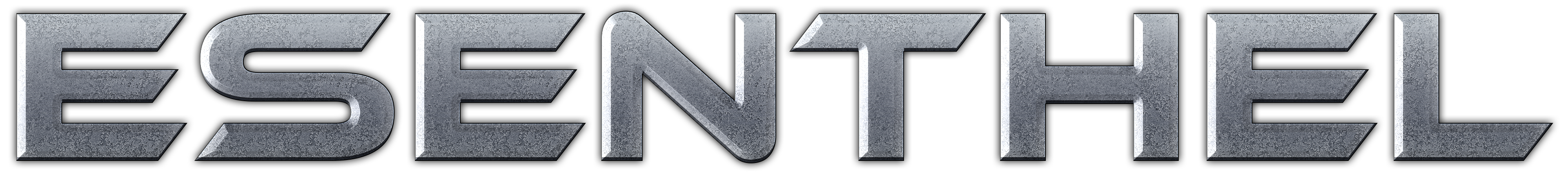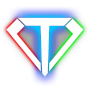About Store Forum Documentation ContactHow to make basic pong Author Message
AlanGhalanMember

 Post: #1 How to make basic pong I want to make a pong game in Esenthel Engine 2.0 to help teach myself.I have the basic idea, but I can't seem to get it working.Here is what I have so far. bool Update() { if(Kb.bp(KB_ESC))return false; point.x-=Time.d(); point.y-=Time.d(); if(point.x <= -1.331)point.x+=Time.d()*10; if(point.x >= 1.331)point.x-=Time.d()*10; if(point.y <= -1)point.y+=Time.d()*10; if(point.y >= 1)point.y-=Time.d()*10; return true; } How can I make a working pong game? Thanks in advance.
01-22-2014 04:12 AM
EsenthelTottelPost: #3 RE: How to make basic pong Hi there, Pong is a great little game to start with! I'll just assume that you're pretty new to programming. - As Esenthel said, use 2 vectors to control your ball. "Position", is obviously the position of the ball. And "Velocity" is the displacement that you'll add to the Position, each frame. If you don't know about vector math, I would strongly recommend to look into that. - The great thing about pong is that the collisions (with level + paddle) are axis-alligned. This means that every object is rectangular and alligned to 0/90/180/.. degree angles. Because of this, you don't have to use "real" physics calculations, but you can simply calculate a collision like so (in pseudo code, I leave the actual programming up to you). Also, don't use values like -1.331, .. Instead use D.x()/D.w() and D.y()/D.h() to get the width and height of the screen. if(BallPos.x <= LeftSideOfScreen || BallPos.x >= RightSideOfScreen) Flip horizontal movement; if(BallPos.y <= TopSideOfScreen || BallPos.y >= BottomSideOfScreen) Flip vertical movement; The great thing about using Pos and Vel, is that you don't need be fiddling with the position. You change the velocity vector. This will define where the position will be on the next frame. For the code above, keep in mind that the BallPos is the center of the ball. You'll also need to take the radius into account. When you have that bouncing around your screen, it's the same thing for collision with paddles. Then you can also add a rectangular area on each side. If you're in this area (again, same code), you get a point! Good luck!